A J   G a l l e r y  - F i n e   C h i n e s e   A r t   &   A n t i q u e s

155 E 71 Street New York NY 10021            Tel: 212 585 2675    www.ajgallery.com    email: aj@ajgallery.com

Chronology of China

Neolithic Period........6500-1700BC

Xia Dynasty........2100-1600BC

Shang Dynasty........1600-1100BC

Zhou Dynasty........1100-256BC

Spring & Autumn........770-475BC

Warring States........475-221BC

Qin Dynasty........221-206BC

Three Kingdoms.........220-265

Jin Dynasty........265-420

Southern Dynasty........420-581

Northern Dynasty........420-581

Sui Dynasty........581-618

Tang Dynasty........618-907

Liao Dynasty........907-960

Song Dynasty........960-1279

Jin Dynasty........1115-1234

Yuan Dynasty........1279-1368

Ming Dynasty........1368-1644

Qing Dynasty........1644-1911

Republic of China........1911-1949

The People's Republic of China........1949-today

See our cloisonnes >>    See our jade carvings >>    See our Chinese paintings >>    See our potteries >>    See our porcelains >>

*            *            *

Our cloisonne collections     Click image to see details    Call us for more other pieces: 212-585-2675   email: aj@ajgallery.com

C1                      C2                      C3                     C4                      C5                      C6                      C7

Our jade carving collections     Click image to see details    Call us for more other pieces: 212-585-2675   email: aj@ajgallery.com

J1                      J2                        J3                       J4                      J5

Our painting collections     Click image to see details    Call us for more other pieces: 212-585-2675   email: aj@ajgallery.com

D1                      D2                      D3                       D4                     D5                      D6                      D7

Our pottery collections     Click image to see details    Call us for more other pieces: 212-585-2675   email: aj@ajgallery.com

A1                      A2                      A4                      A5                      A6                     A7                      A8

Our porcelain collections     Click image to see details    Call us for more other pieces: 212-585-2675   email: aj@ajgallery.com

P1                      P2                       P3                      P4                      P5                      P6

*        *        *

Our pottery collections     Call us for more other pieces: 212-585-2675   email: aj@ajgallery.com                << back to top >>

A J   G a l l e r y  - F i n e   C h i n e s e   A r t   &   A n t i q u e s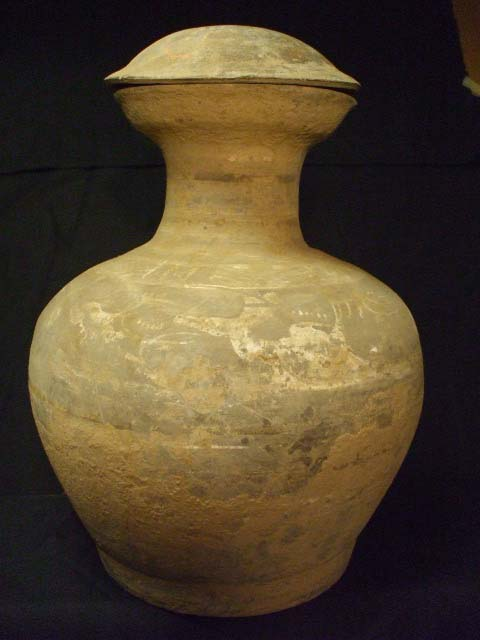a11    << go back >>

A J   G a l l e r y  - F i n e   C h i n e s e   A r t   &   A n t i q u e s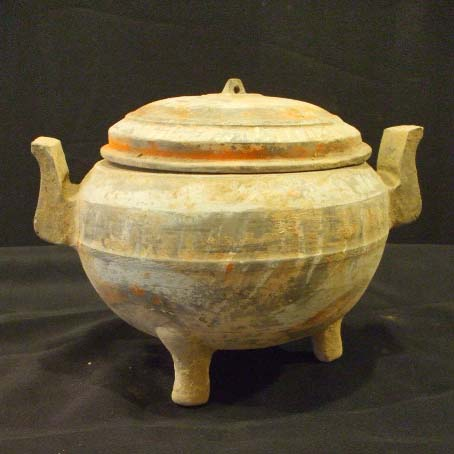a12    << go back >>

A J   G a l l e r y  - F i n e   C h i n e s e   A r t   &   A n t i q u e s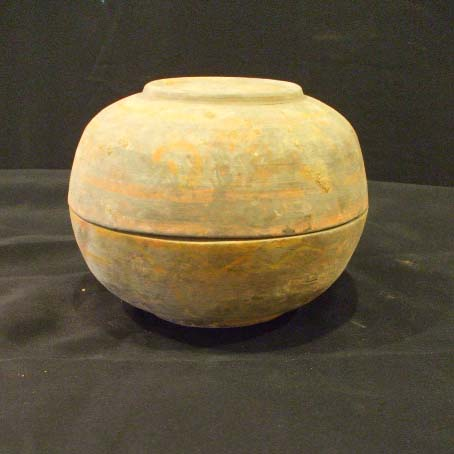a13    << go back >>

A J   G a l l e r y  - F i n e   C h i n e s e   A r t   &   A n t i q u e s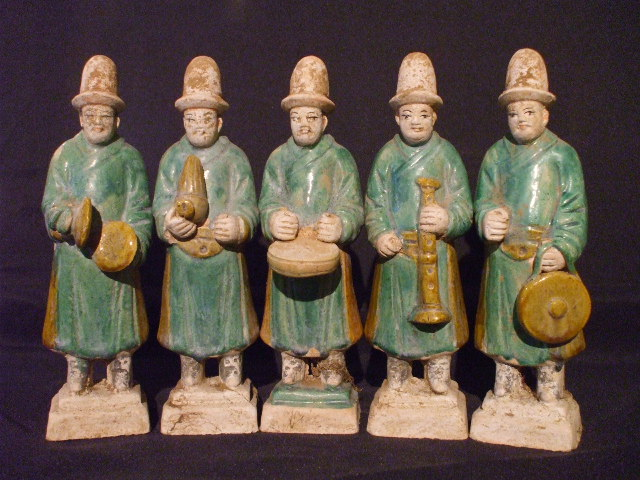a21    << go back >>

A J   G a l l e r y  - F i n e   C h i n e s e   A r t   &   A n t i q u e s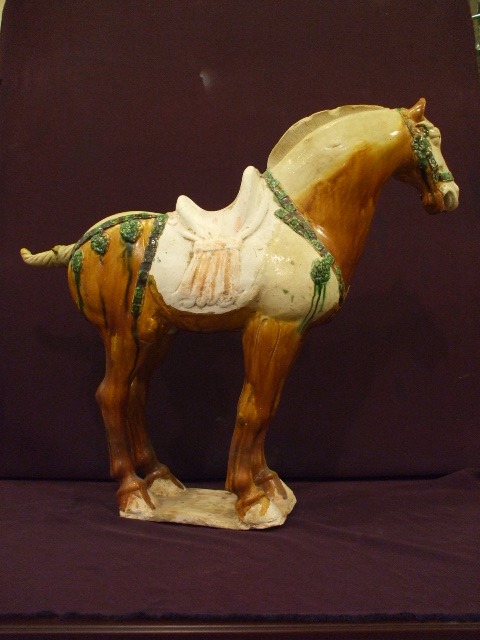a31    << go back >>

A J   G a l l e r y  - F i n e   C h i n e s e   A r t   &   A n t i q u e s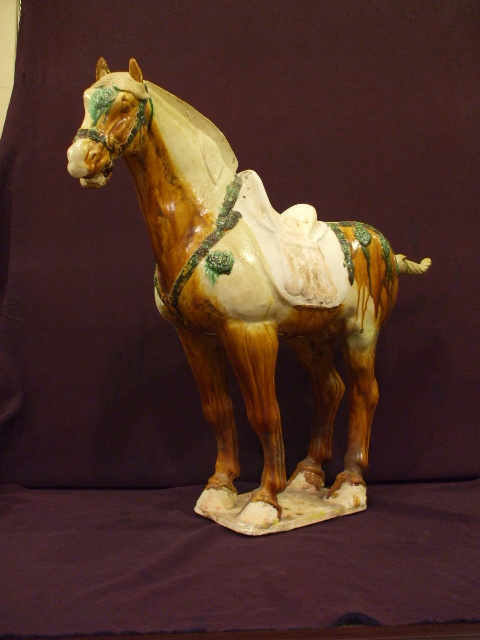a33    << go back >>

A J   G a l l e r y  - F i n e   C h i n e s e   A r t   &   A n t i q u e s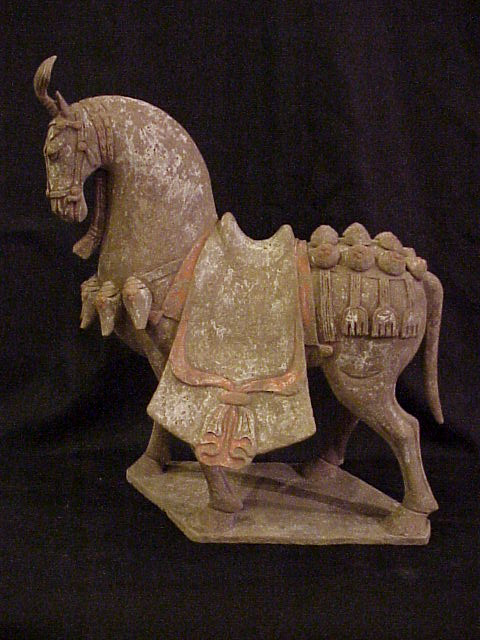a41    << go back >>

A J   G a l l e r y  - F i n e   C h i n e s e   A r t   &   A n t i q u e s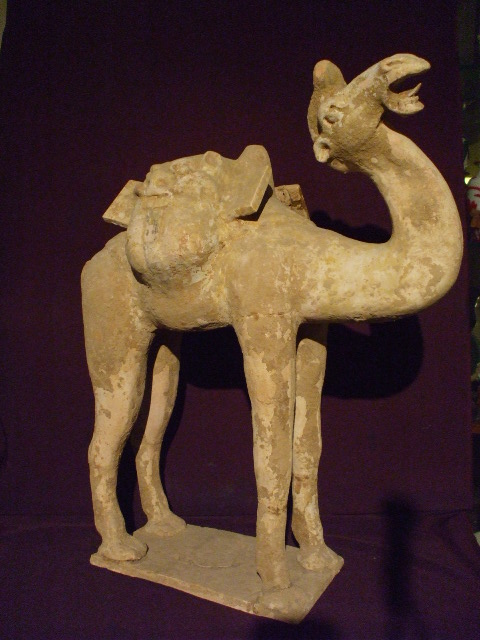a51    << go back >>

A J   G a l l e r y  - F i n e   C h i n e s e   A r t   &   A n t i q u e s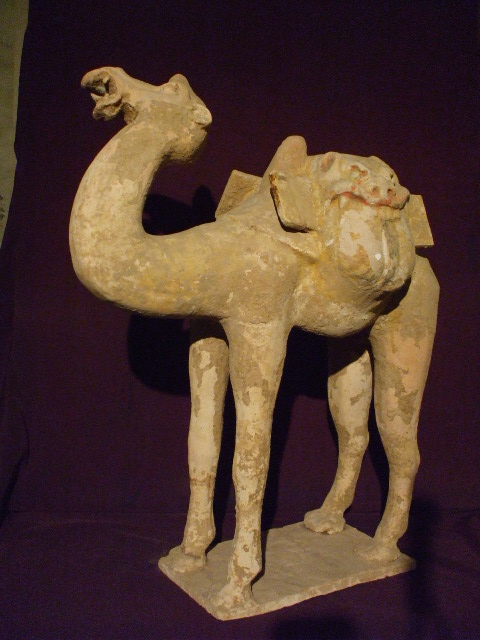a52    << go back >>

A J   G a l l e r y  - F i n e   C h i n e s e   A r t   &   A n t i q u e s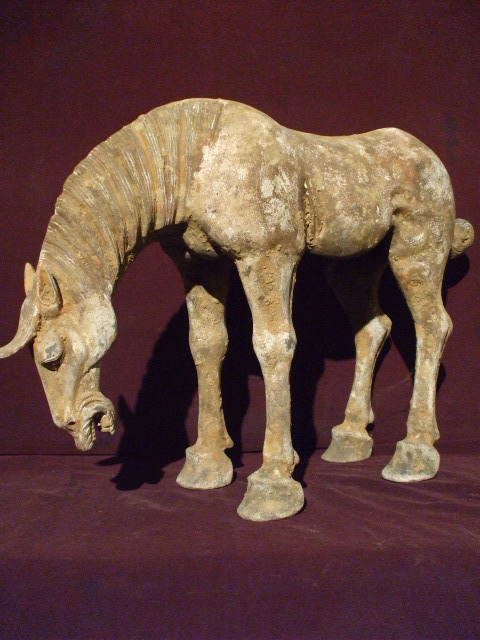a61    << go back >>

A J   G a l l e r y  - F i n e   C h i n e s e   A r t   &   A n t i q u e s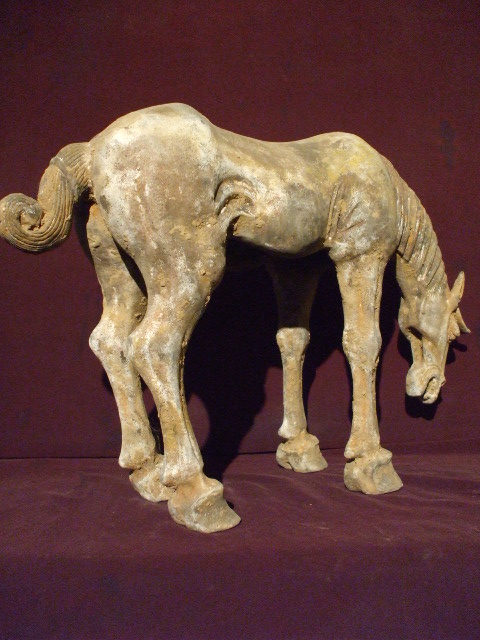a62    << go back >>

A J   G a l l e r y  - F i n e   C h i n e s e   A r t   &   A n t i q u e s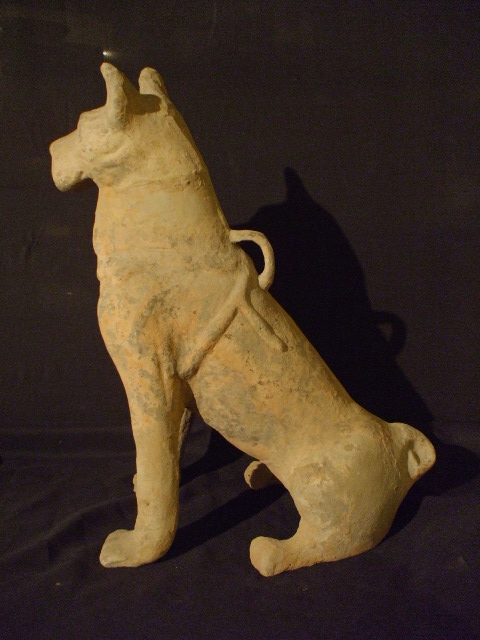a71    << go back >>

A J   G a l l e r y  - F i n e   C h i n e s e   A r t   &   A n t i q u e s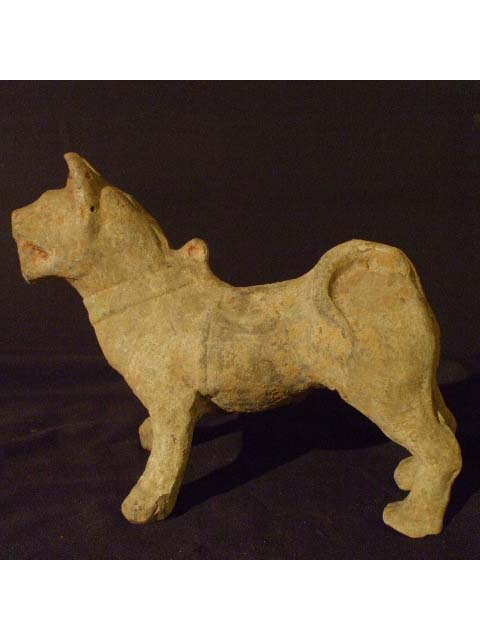a72    << go back >>

A J   G a l l e r y  - F i n e   C h i n e s e   A r t   &   A n t i q u e s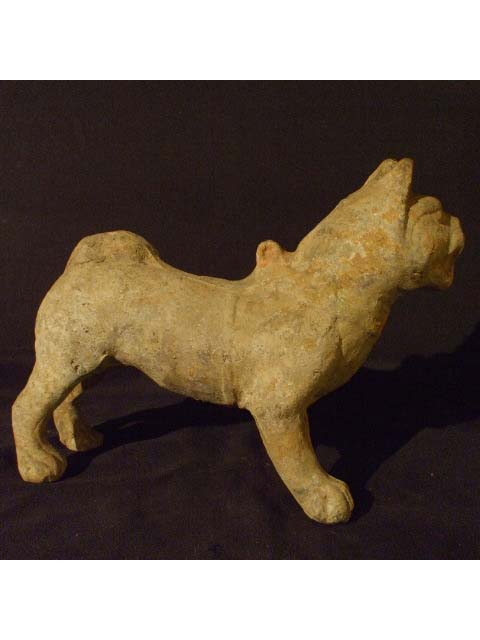a73    << go back >>

A J   G a l l e r y  - F i n e   C h i n e s e   A r t   &   A n t i q u e s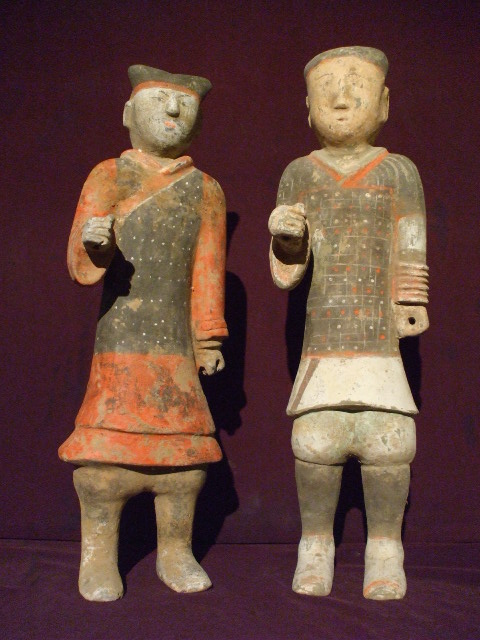a81    << go back >>

A J   G a l l e r y  - F i n e   C h i n e s e   A r t   &   A n t i q u e s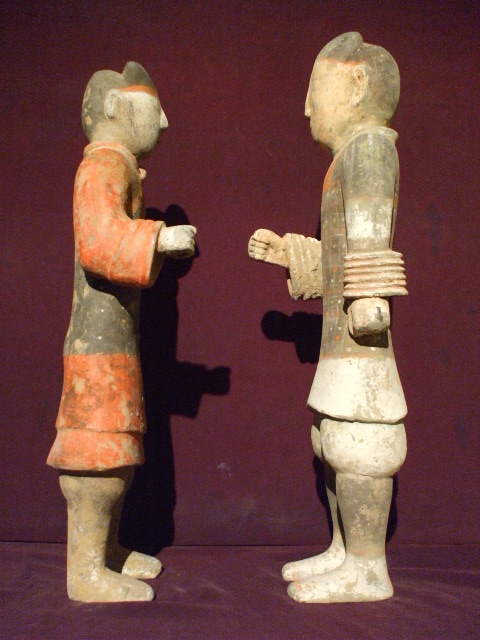a82    << go back >>

*        *        *

A J   G a l l e r y  - F i n e   C h i n e s e   A r t   &   A n t i q u e s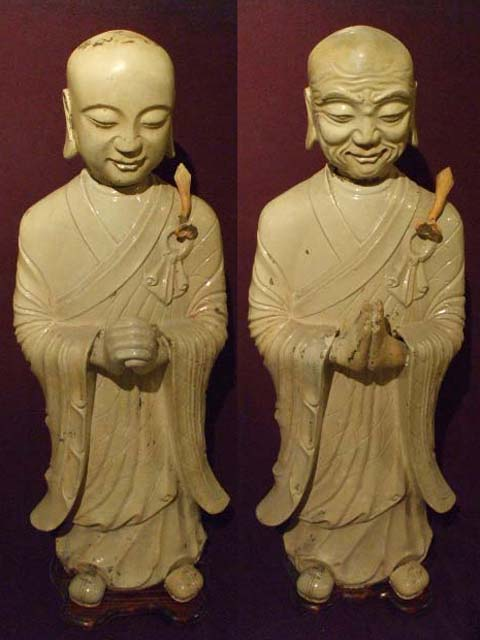b11

*        *        *

Our cloisonne collections     Call us for more other pieces: 212-585-2675   email: aj@ajgallery.com                << back to top >>

A J   G a l l e r y  - F i n e   C h i n e s e   A r t   &   A n t i q u e s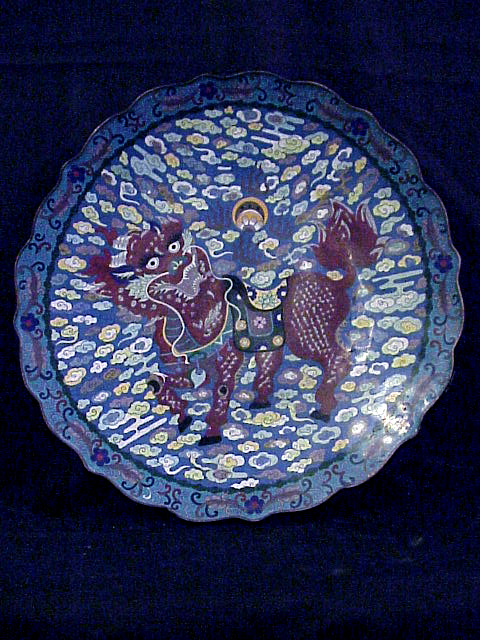c11    << go back >>

A J   G a l l e r y  - F i n e   C h i n e s e   A r t   &   A n t i q u e s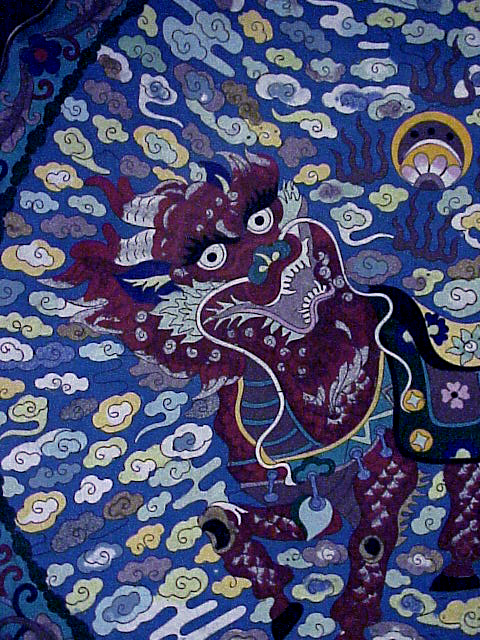A J   G a l l e r y  - F i n e   C h i n e s e   A r t   &   A n t i q u e s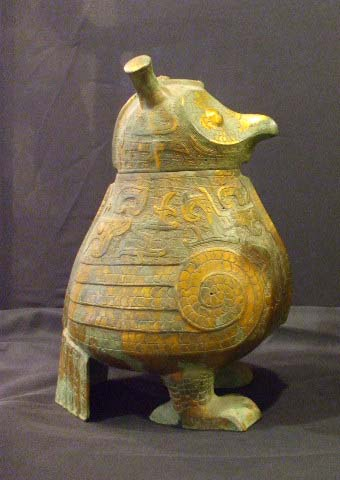c21    << go back >>

A J   G a l l e r y  - F i n e   C h i n e s e   A r t   &   A n t i q u e s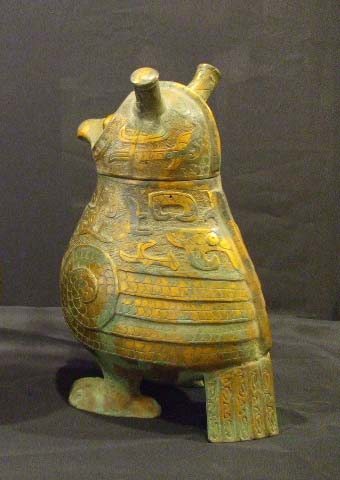A J   G a l l e r y  - F i n e   C h i n e s e   A r t   &   A n t i q u e s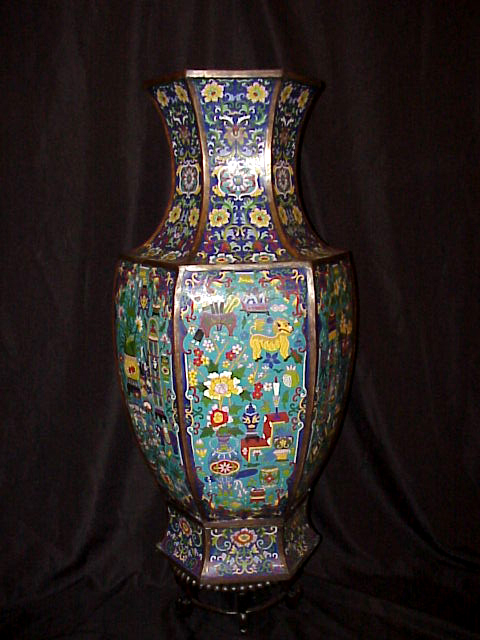c31    << go back >>

A J   G a l l e r y  - F i n e   C h i n e s e   A r t   &   A n t i q u e s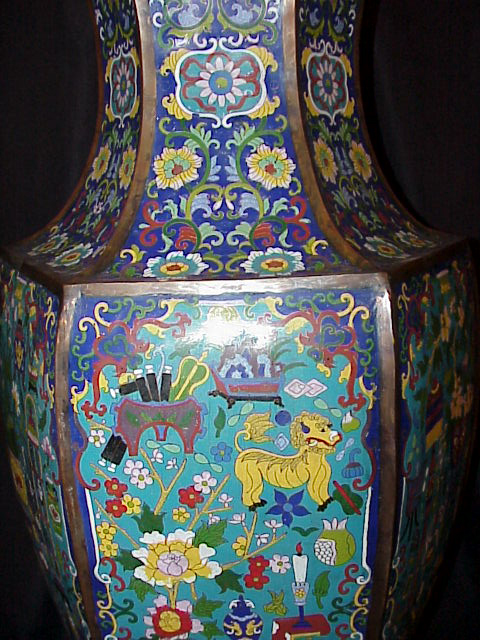A J   G a l l e r y  - F i n e   C h i n e s e   A r t   &   A n t i q u e s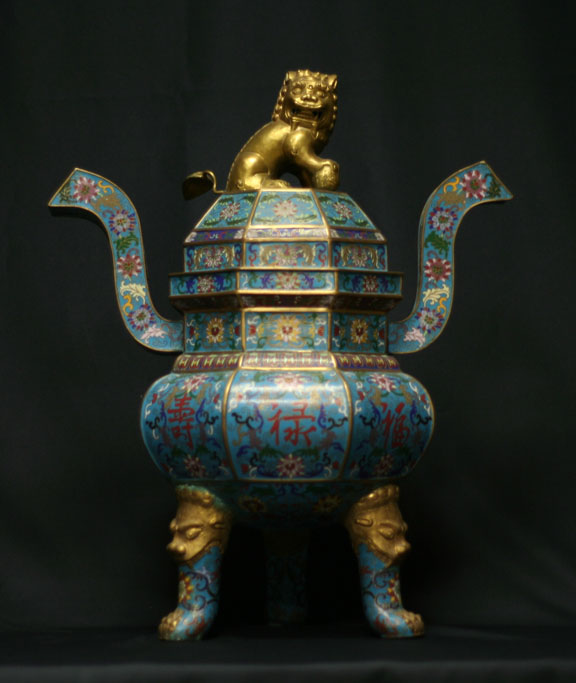c41    << go back >>

A J   G a l l e r y  - F i n e   C h i n e s e   A r t   &   A n t i q u e s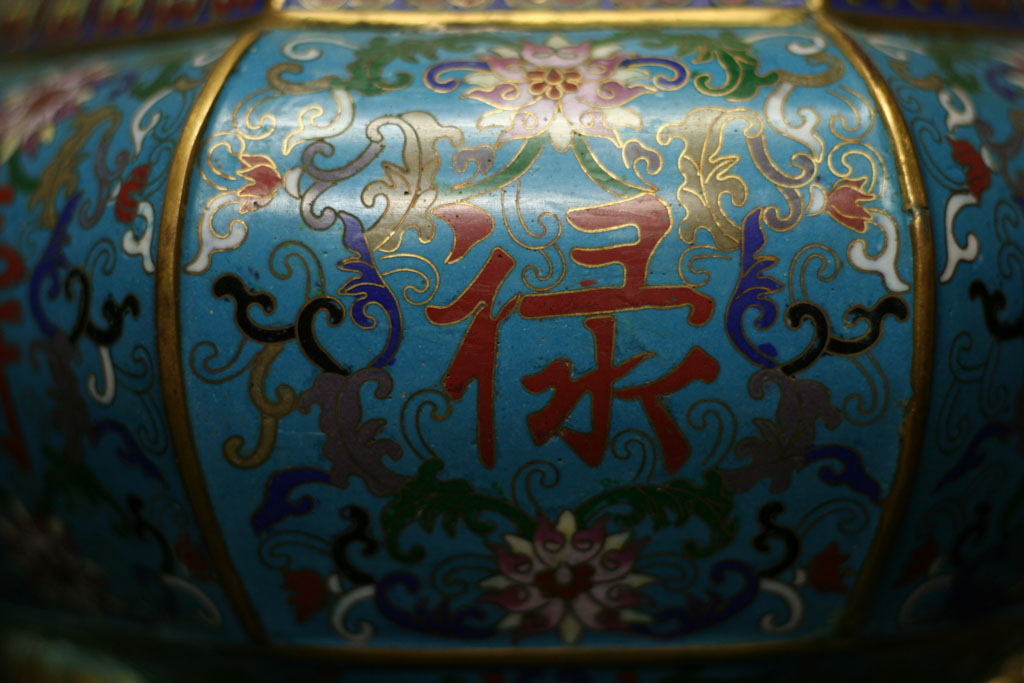A J   G a l l e r y  - F i n e   C h i n e s e   A r t   &   A n t i q u e s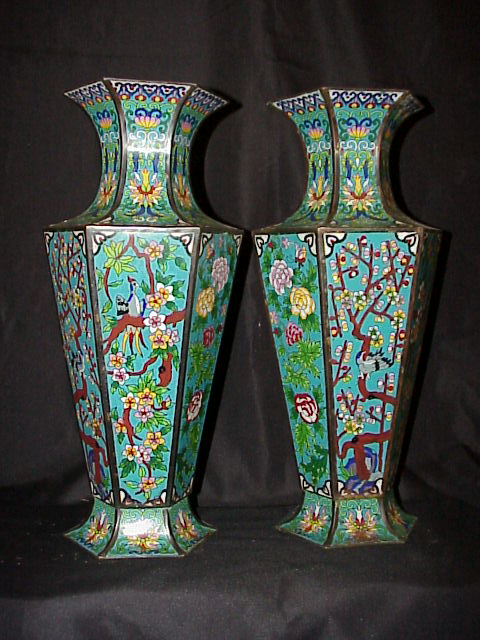c51    << go back >>

A J   G a l l e r y  - F i n e   C h i n e s e   A r t   &   A n t i q u e s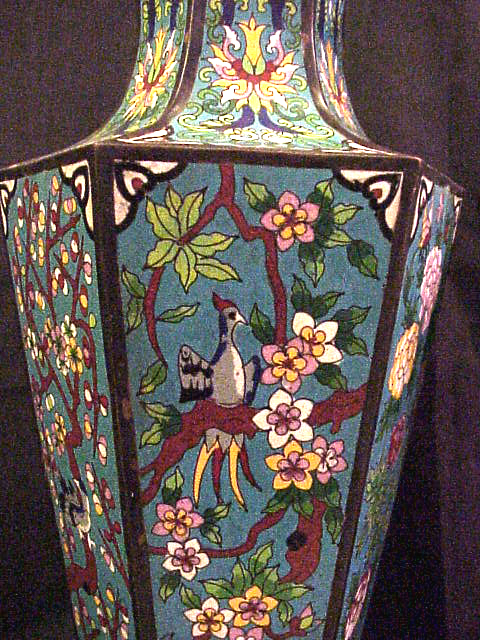A J   G a l l e r y  - F i n e   C h i n e s e   A r t   &   A n t i q u e s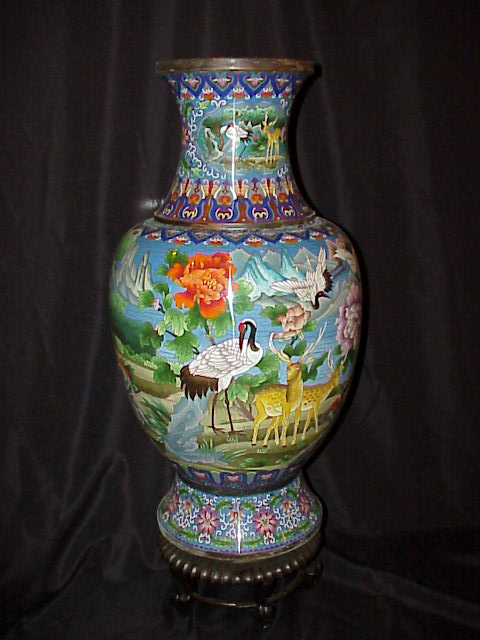c61    << go back >>

A J   G a l l e r y  - F i n e   C h i n e s e   A r t   &   A n t i q u e s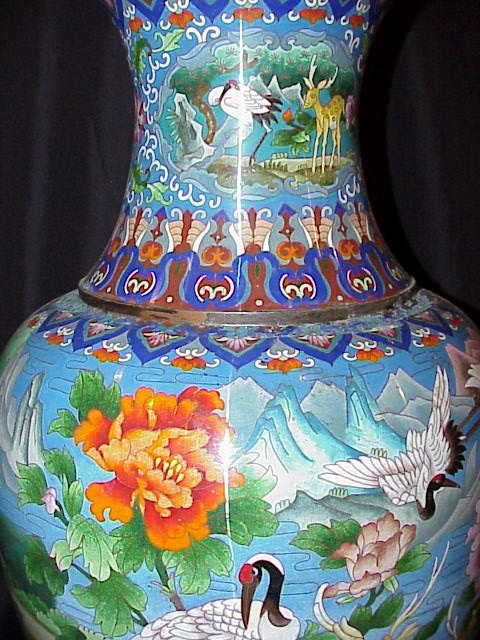A J   G a l l e r y  - F i n e   C h i n e s e   A r t   &   A n t i q u e s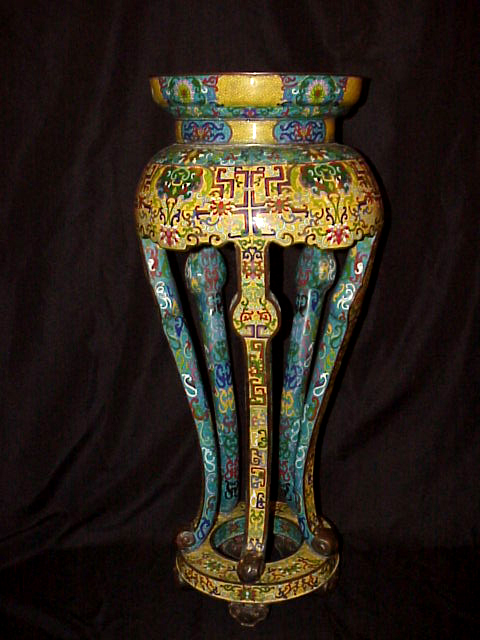c71    << go back >>

A J   G a l l e r y  - F i n e   C h i n e s e   A r t   &   A n t i q u e s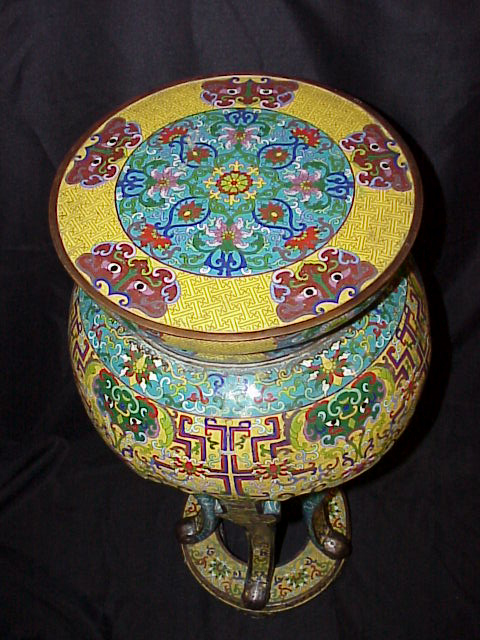*        *        *

Our painting collections     Call us for more other pieces: 212-585-2675   email: aj@ajgallery.com                << back to top >>

A J   G a l l e r y  - F i n e   C h i n e s e   A r t   &   A n t i q u e s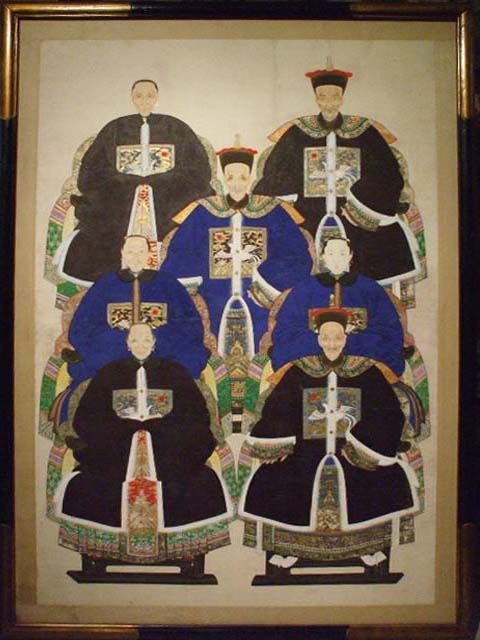d11    << go back >>

A J   G a l l e r y  - F i n e   C h i n e s e   A r t   &   A n t i q u e s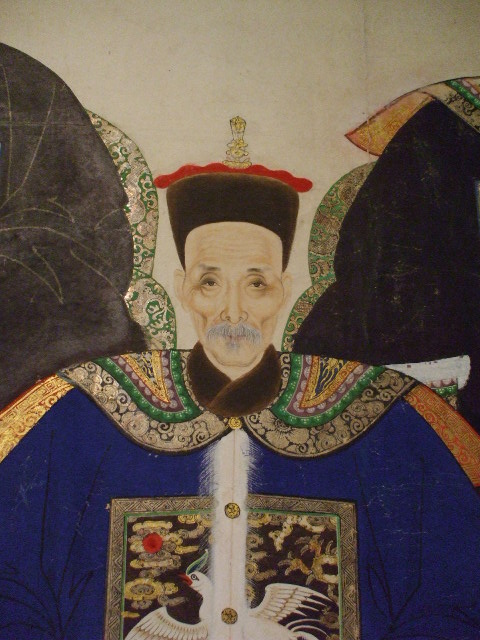A J   G a l l e r y  - F i n e   C h i n e s e   A r t   &   A n t i q u e s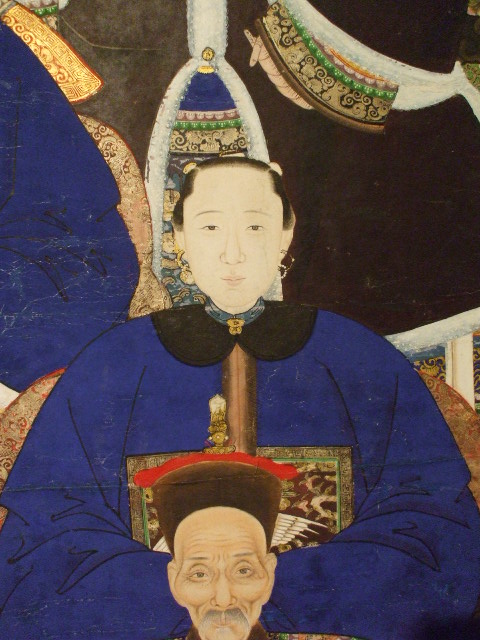A J   G a l l e r y  - F i n e   C h i n e s e   A r t   &   A n t i q u e s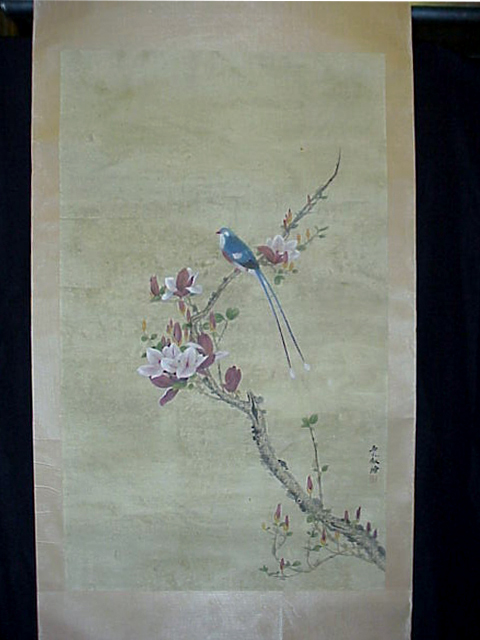d21    << go back >>

A J   G a l l e r y  - F i n e   C h i n e s e   A r t   &   A n t i q u e s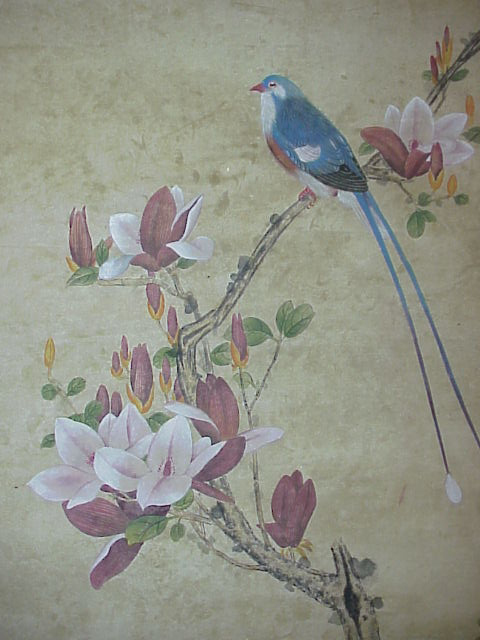A J   G a l l e r y  - F i n e   C h i n e s e   A r t   &   A n t i q u e s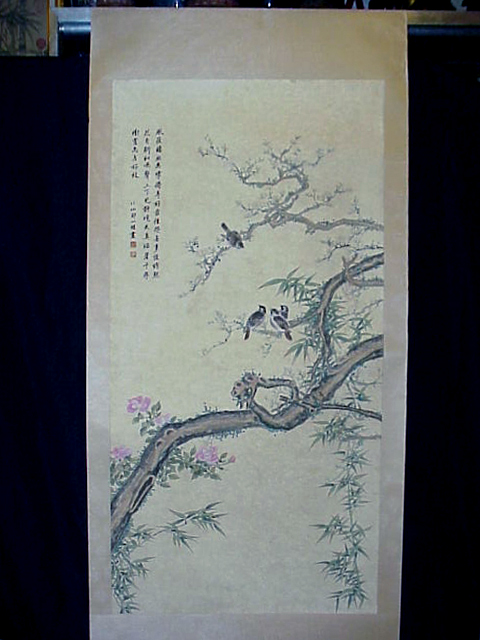d31    << go back >>

A J   G a l l e r y  - F i n e   C h i n e s e   A r t   &   A n t i q u e s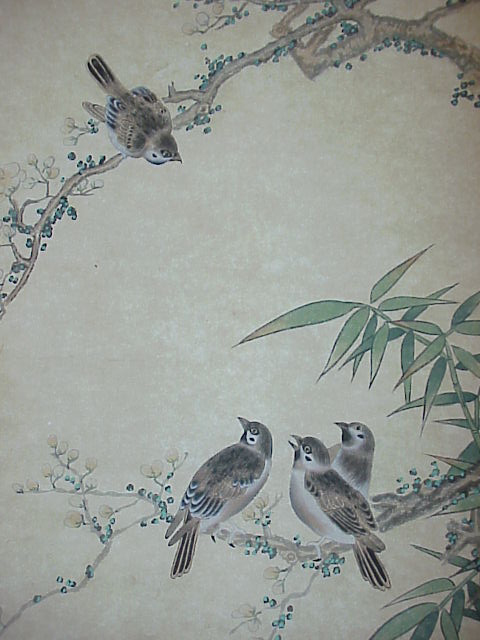A J   G a l l e r y  - F i n e   C h i n e s e   A r t   &   A n t i q u e s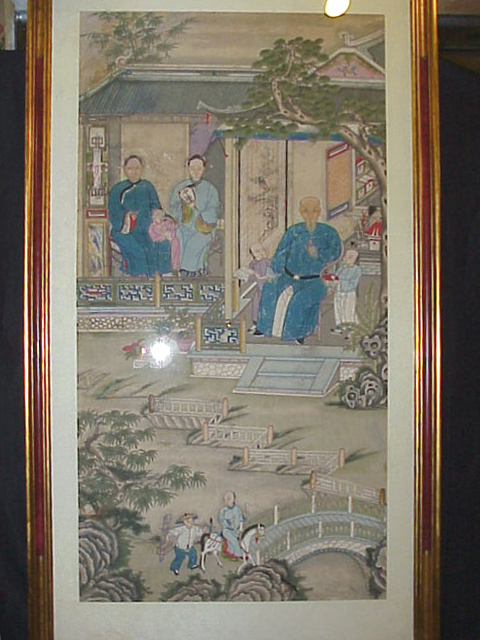d41    << go back >>

A J   G a l l e r y  - F i n e   C h i n e s e   A r t   &   A n t i q u e s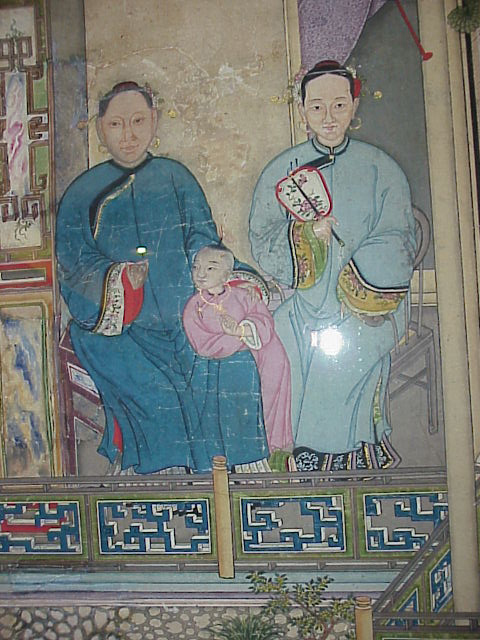A J   G a l l e r y  - F i n e   C h i n e s e   A r t   &   A n t i q u e s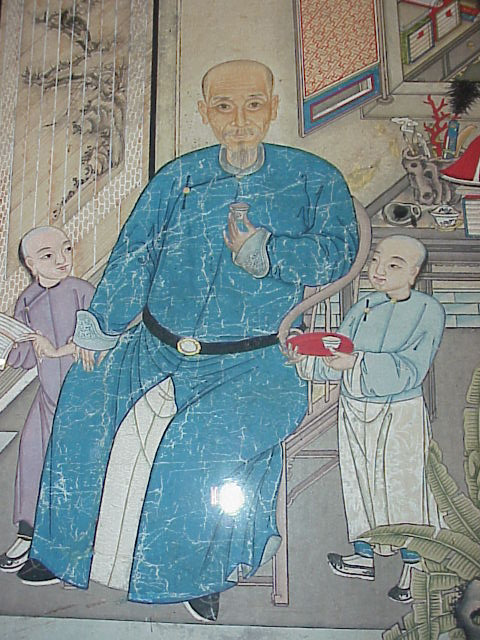A J   G a l l e r y  - F i n e   C h i n e s e   A r t   &   A n t i q u e s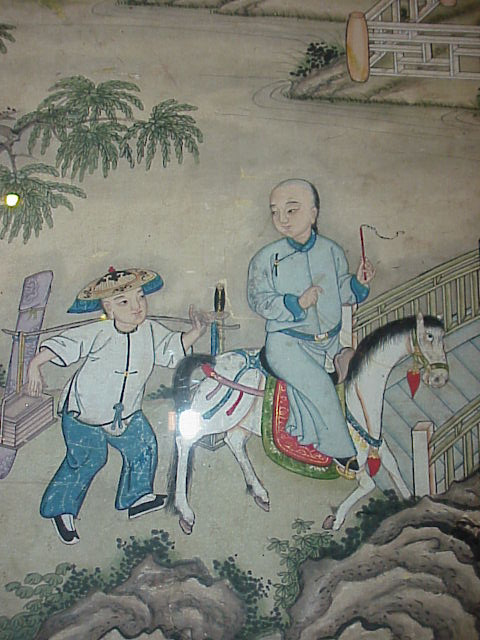A J   G a l l e r y  - F i n e   C h i n e s e   A r t   &   A n t i q u e s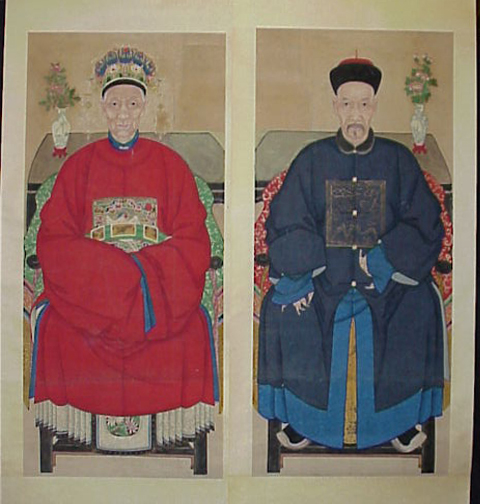d51    << go back >>

A J   G a l l e r y  - F i n e   C h i n e s e   A r t   &   A n t i q u e s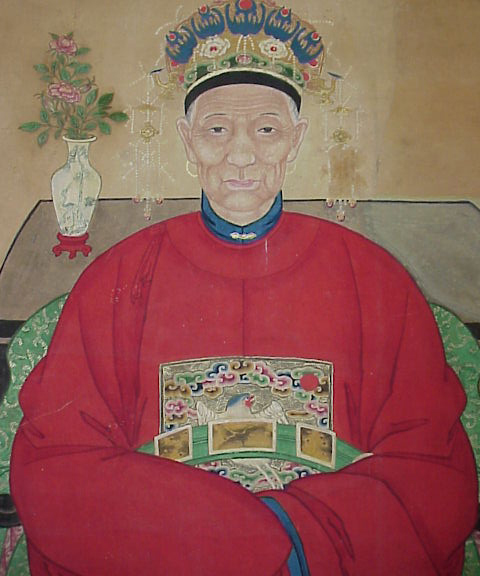A J   G a l l e r y  - F i n e   C h i n e s e   A r t   &   A n t i q u e s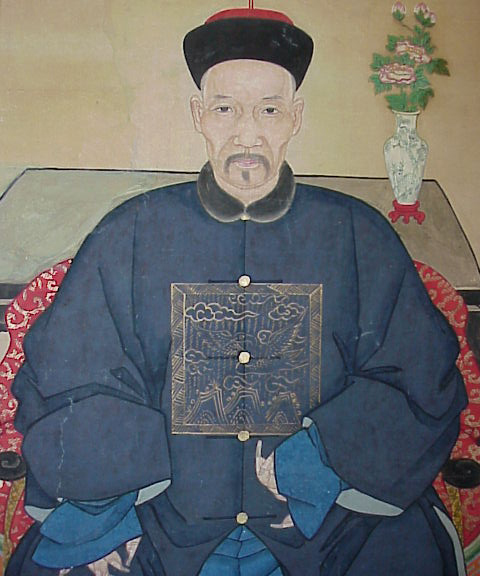A J   G a l l e r y  - F i n e   C h i n e s e   A r t   &   A n t i q u e s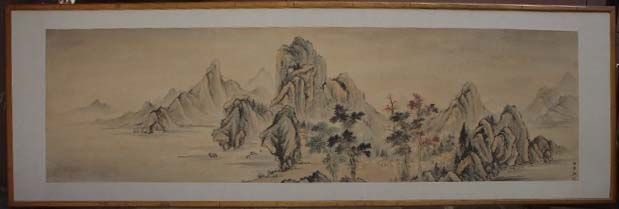d61    << go back >>

A J   G a l l e r y  - F i n e   C h i n e s e   A r t   &   A n t i q u e s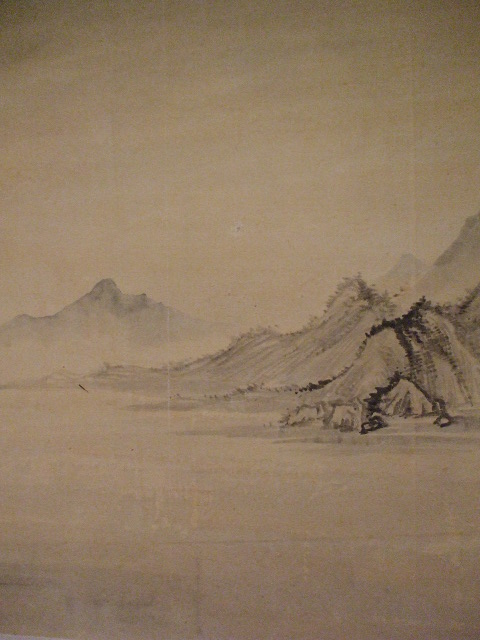A J   G a l l e r y  - F i n e   C h i n e s e   A r t   &   A n t i q u e s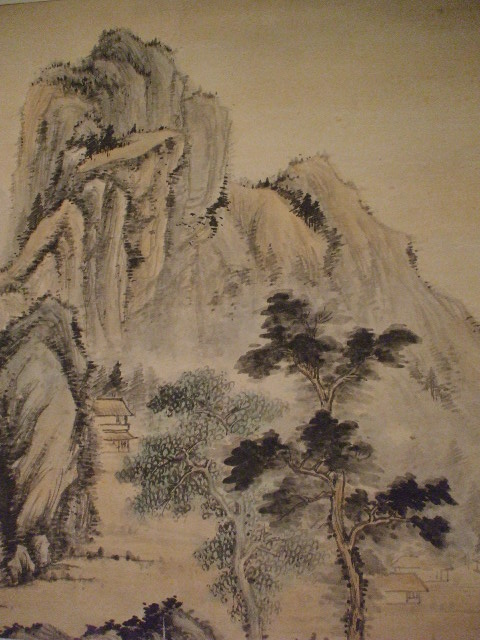A J   G a l l e r y  - F i n e   C h i n e s e   A r t   &   A n t i q u e s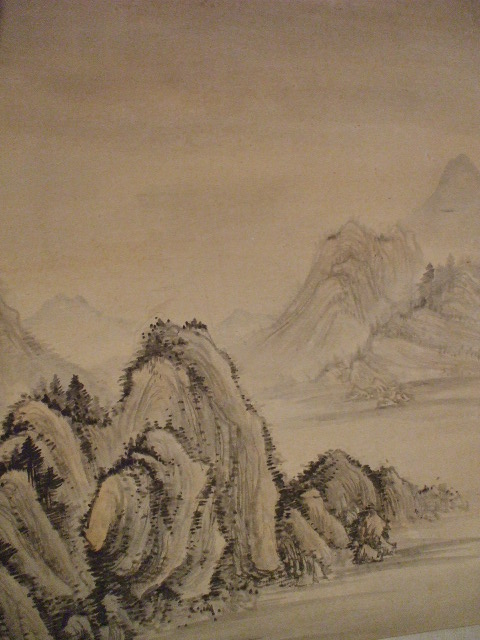A J   G a l l e r y  - F i n e   C h i n e s e   A r t   &   A n t i q u e s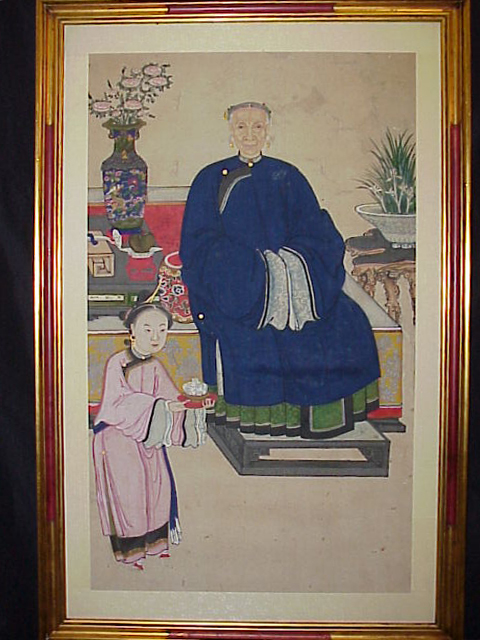d71    << go back >>

A J   G a l l e r y  - F i n e   C h i n e s e   A r t   &   A n t i q u e s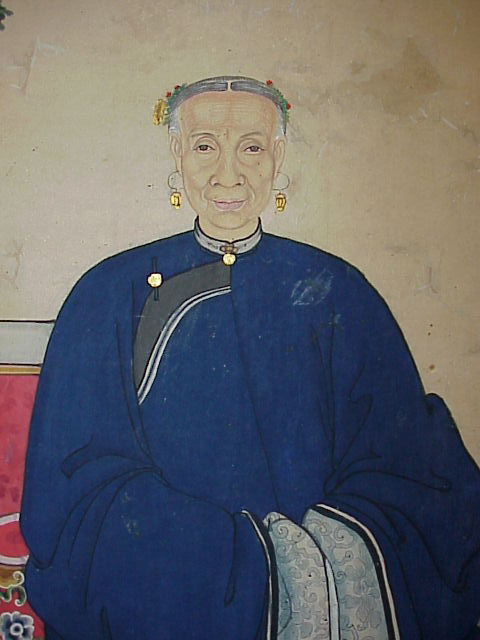A J   G a l l e r y  - F i n e   C h i n e s e   A r t   &   A n t i q u e s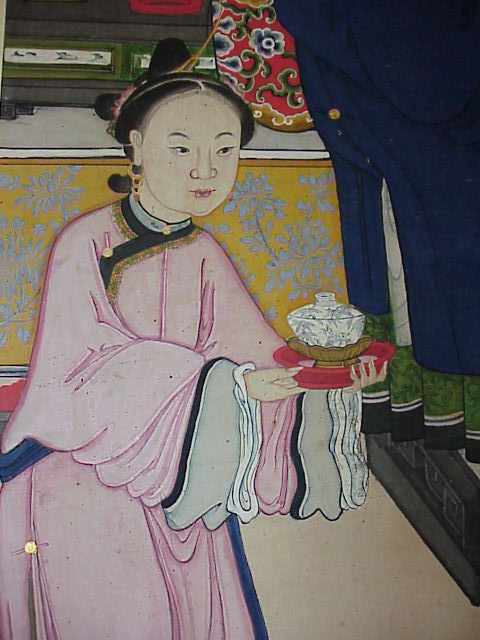*        *        *

A J   G a l l e r y  - F i n e   C h i n e s e   A r t   &   A n t i q u e s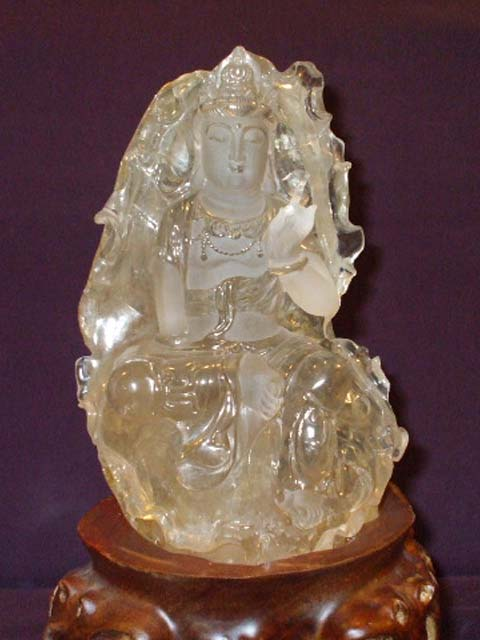j11    << go back >>

A J   G a l l e r y  - F i n e   C h i n e s e   A r t   &   A n t i q u e s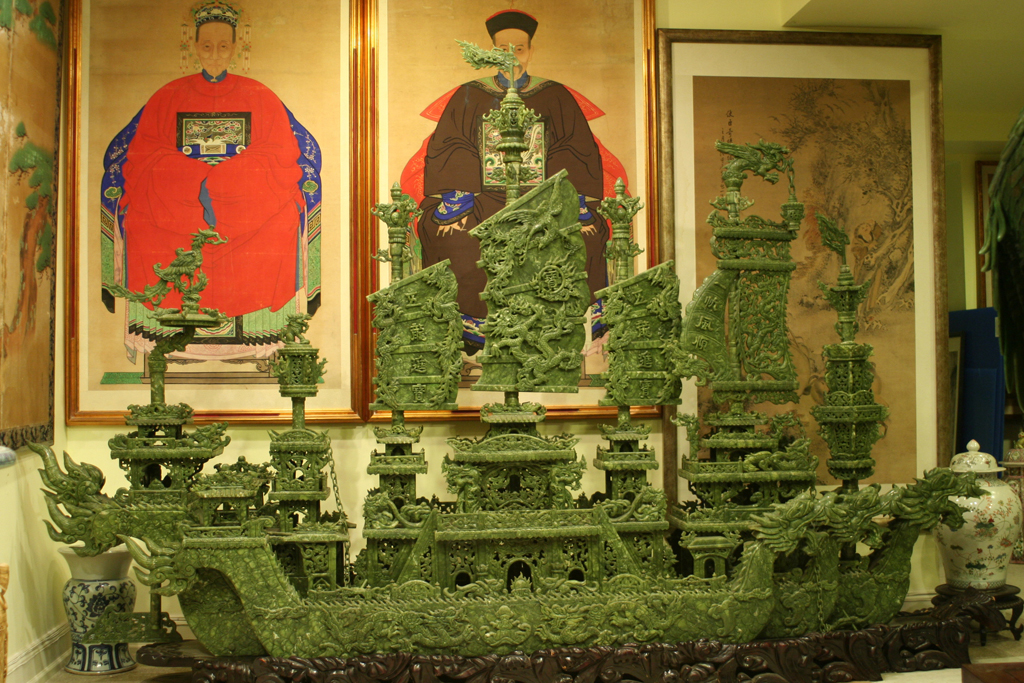j21    << go back >>

A J   G a l l e r y  - F i n e   C h i n e s e   A r t   &   A n t i q u e s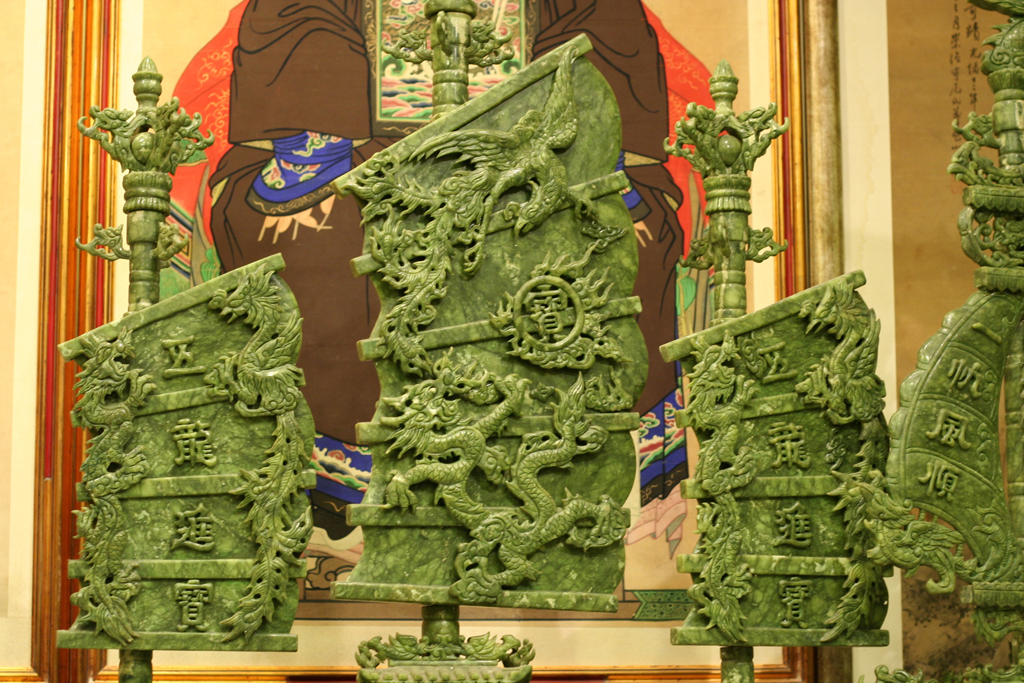A J   G a l l e r y  - F i n e   C h i n e s e   A r t   &   A n t i q u e s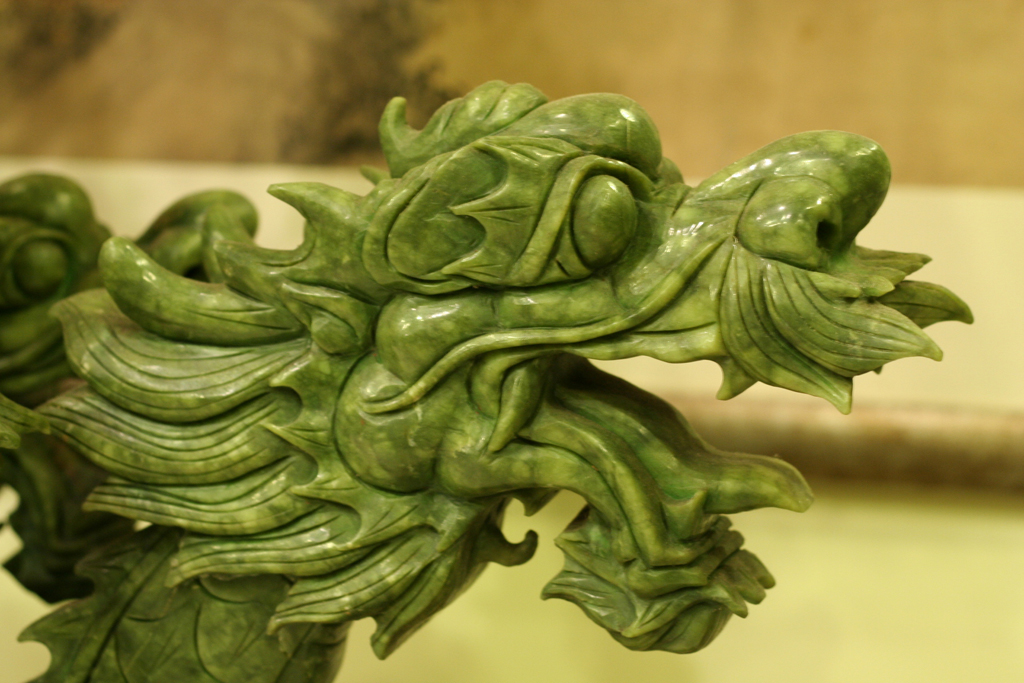A J   G a l l e r y  - F i n e   C h i n e s e   A r t   &   A n t i q u e s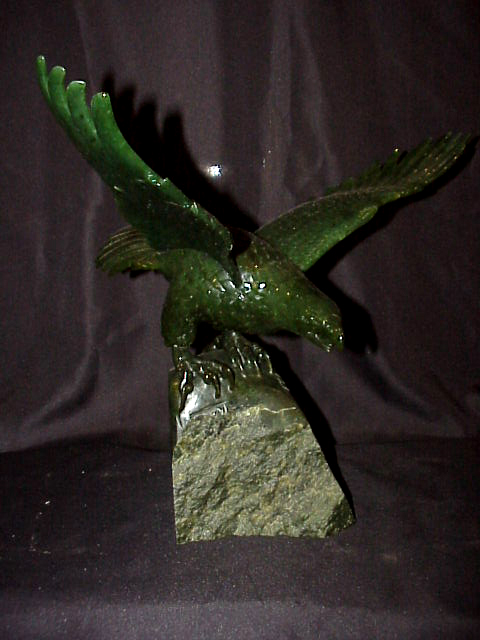j31    << go back >>

A J   G a l l e r y  - F i n e   C h i n e s e   A r t   &   A n t i q u e s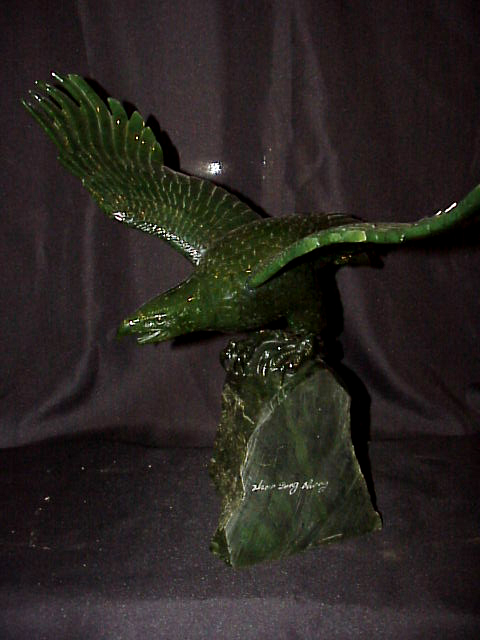A J   G a l l e r y  - F i n e   C h i n e s e   A r t   &   A n t i q u e s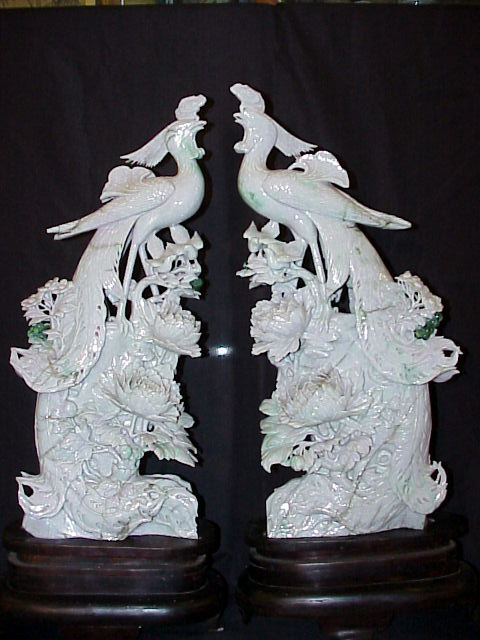j41    << go back >>

A J   G a l l e r y  - F i n e   C h i n e s e   A r t   &   A n t i q u e s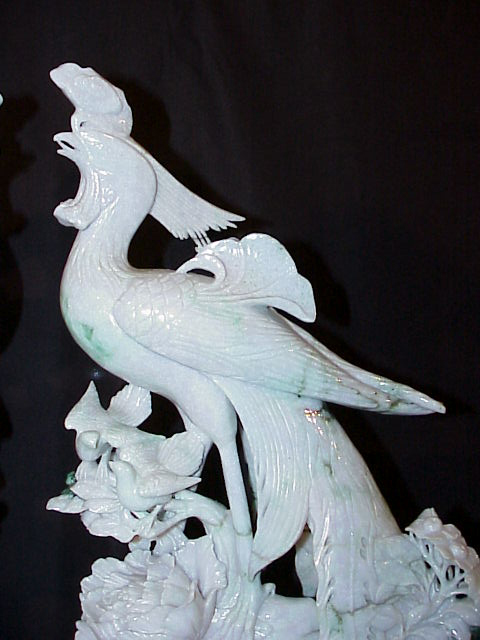A J   G a l l e r y  - F i n e   C h i n e s e   A r t   &   A n t i q u e s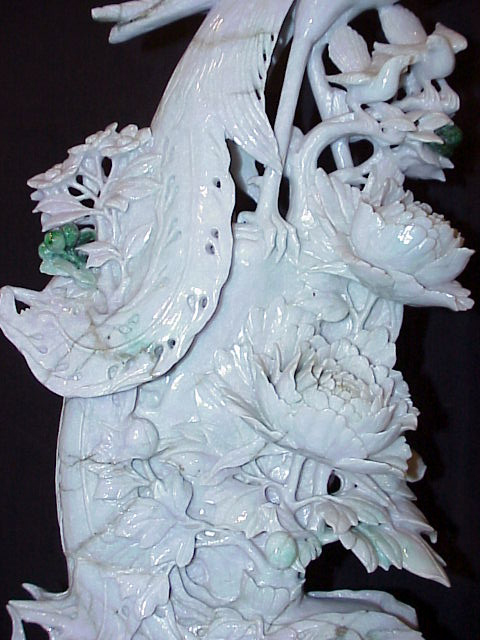A J   G a l l e r y  - F i n e   C h i n e s e   A r t   &   A n t i q u e s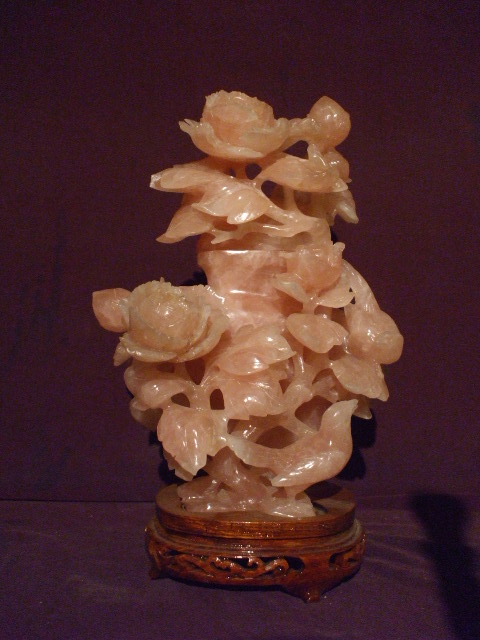j51    << go back >>

A J   G a l l e r y  - F i n e   C h i n e s e   A r t   &   A n t i q u e s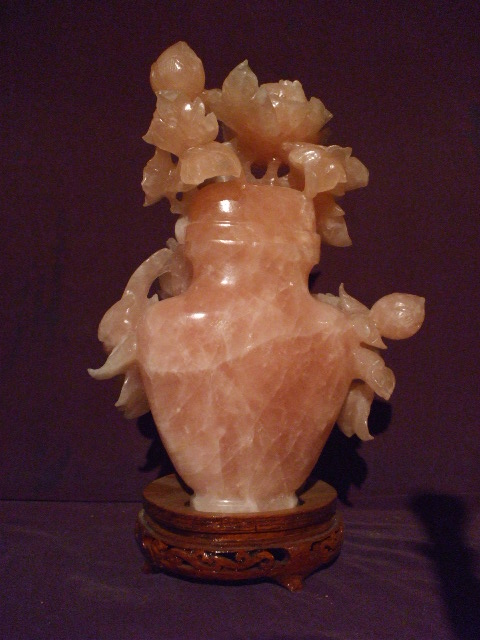*        *        *

Our porcelain collections     Call us for more other pieces: 212-585-2675   email: aj@ajgallery.com                << back to top >>

A J   G a l l e r y  - F i n e   C h i n e s e   A r t   &   A n t i q u e s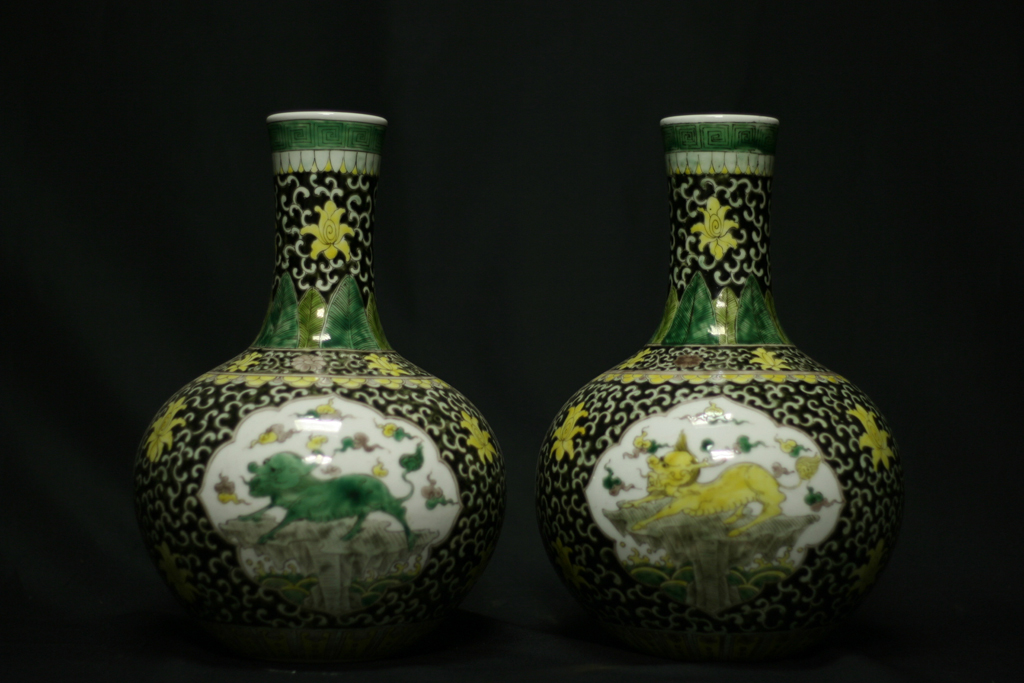p11    << go back >>

A J   G a l l e r y  - F i n e   C h i n e s e   A r t   &   A n t i q u e s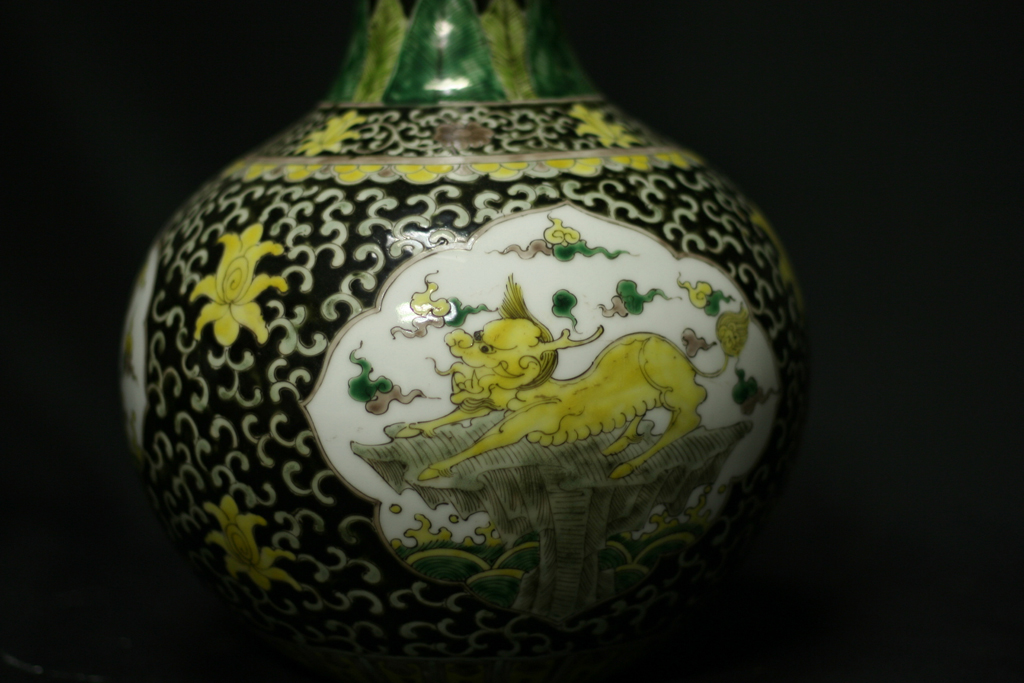A J   G a l l e r y  - F i n e   C h i n e s e   A r t   &   A n t i q u e s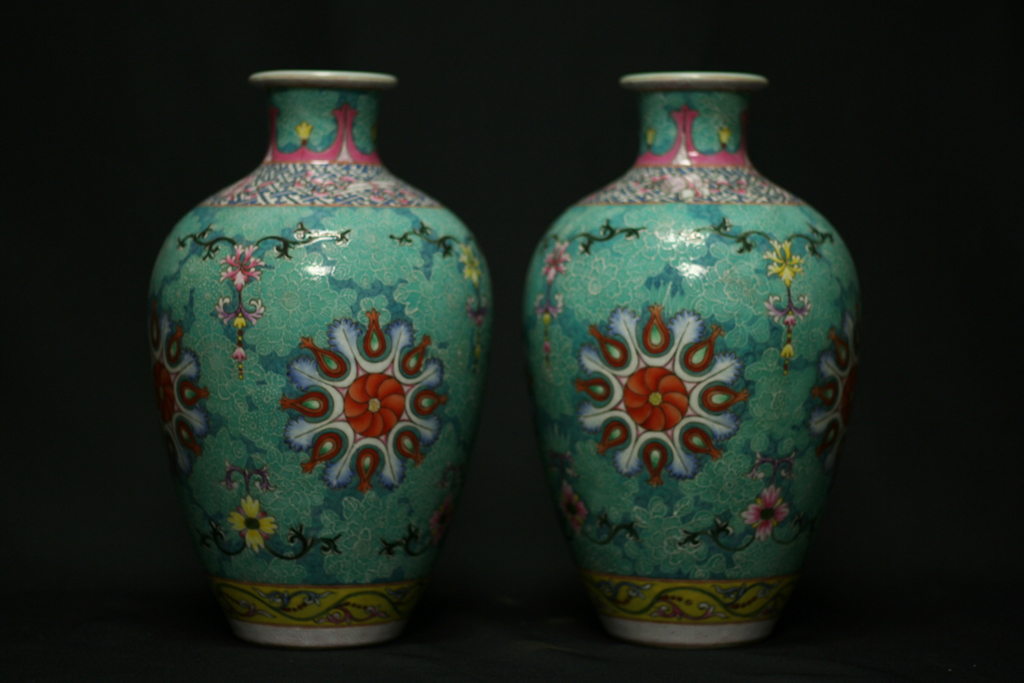p21    << go back >>

A J   G a l l e r y  - F i n e   C h i n e s e   A r t   &   A n t i q u e s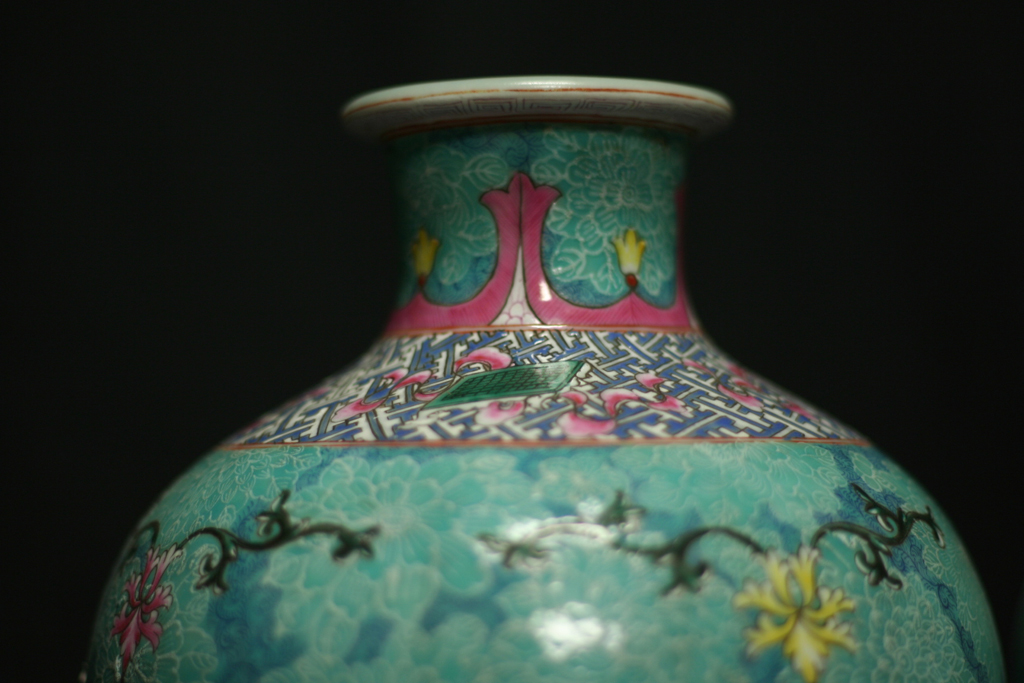A J   G a l l e r y  - F i n e   C h i n e s e   A r t   &   A n t i q u e s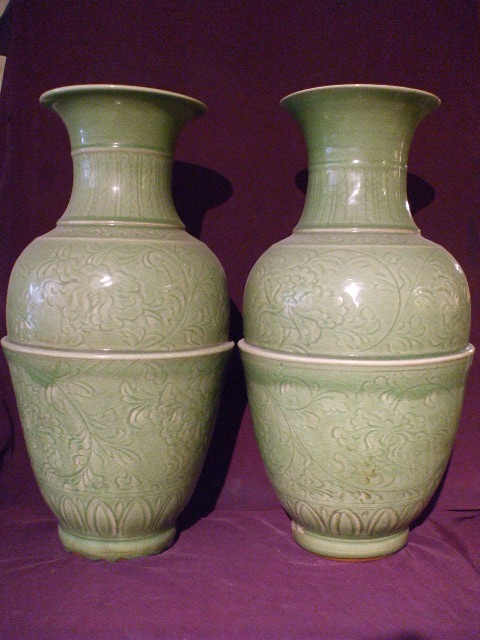p31    << go back >>

A J   G a l l e r y  - F i n e   C h i n e s e   A r t   &   A n t i q u e s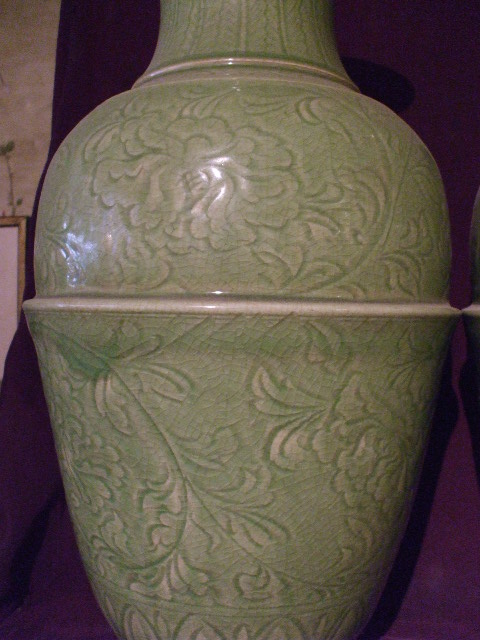A J   G a l l e r y  - F i n e   C h i n e s e   A r t   &   A n t i q u e s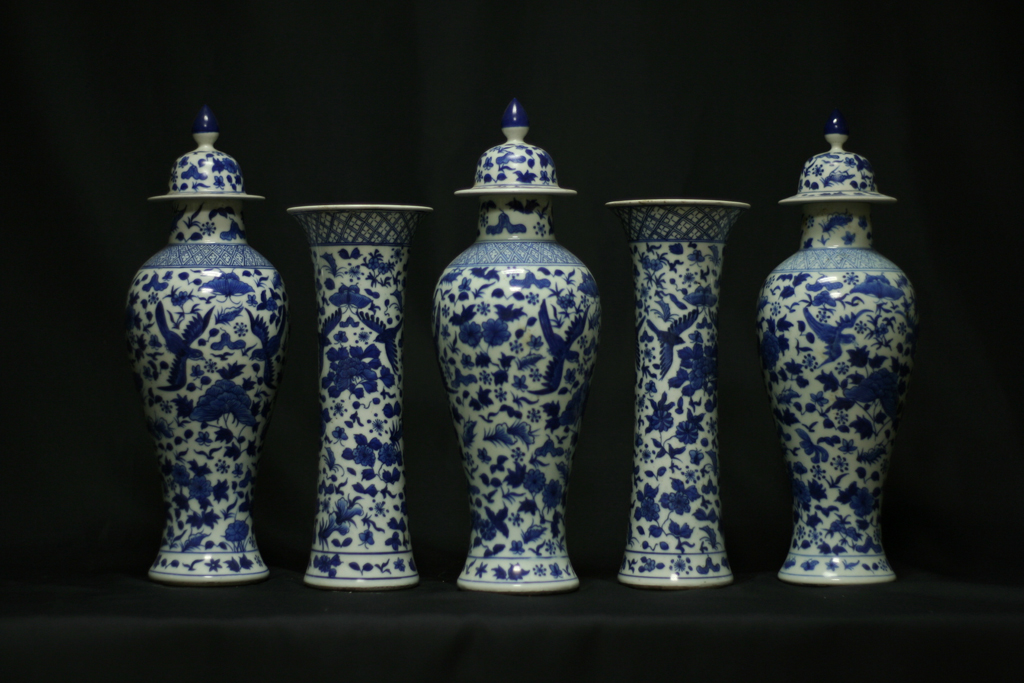p41    << go back >>

A J   G a l l e r y  - F i n e   C h i n e s e   A r t   &   A n t i q u e s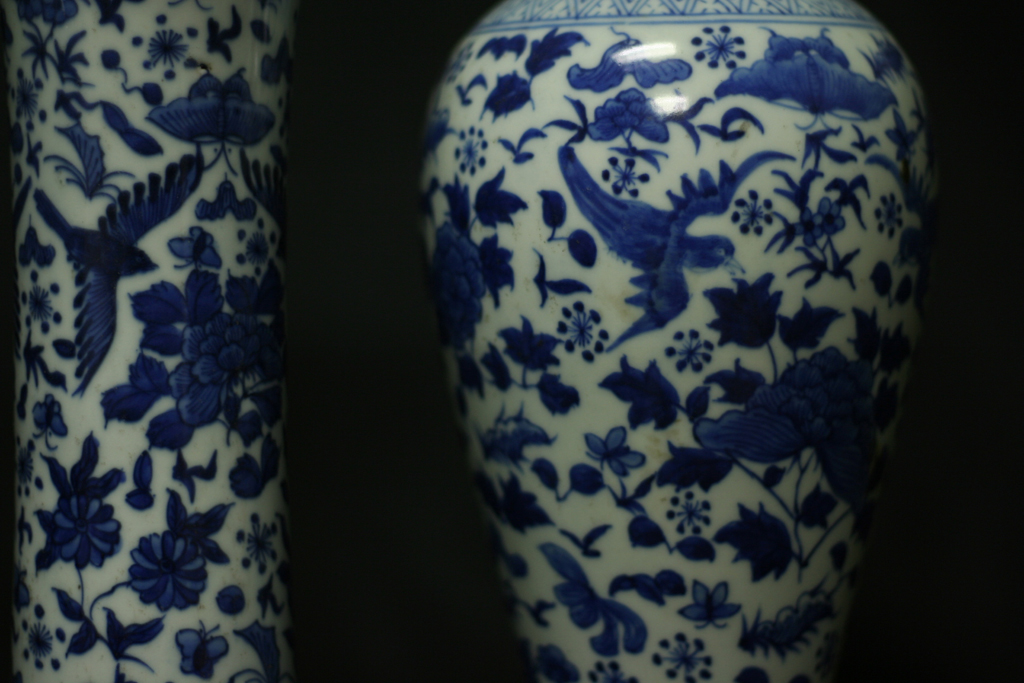A J   G a l l e r y  - F i n e   C h i n e s e   A r t   &   A n t i q u e s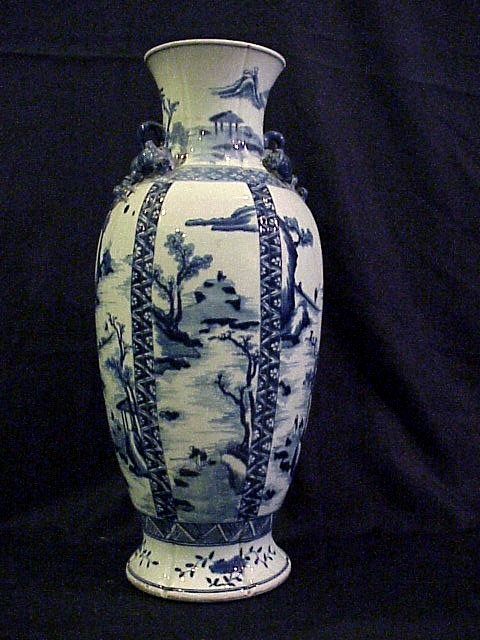p51    << go back >>

A J   G a l l e r y  - F i n e   C h i n e s e   A r t   &   A n t i q u e s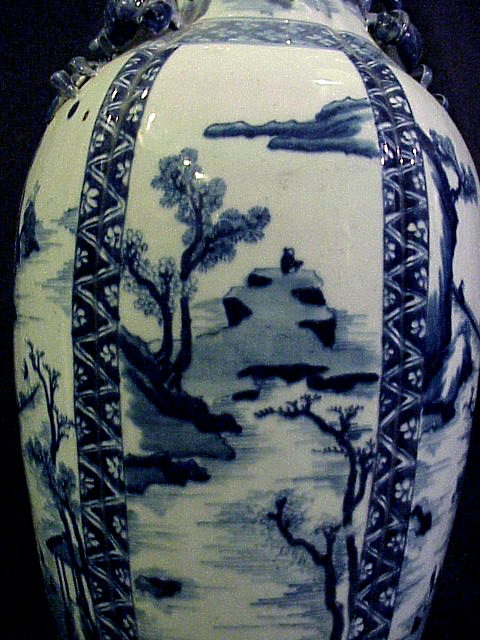A J   G a l l e r y  - F i n e   C h i n e s e   A r t   &   A n t i q u e s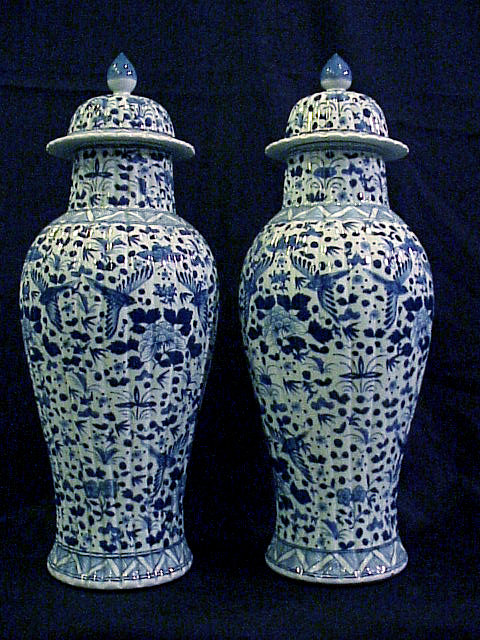p61    << go back >>

A J   G a l l e r y  - F i n e   C h i n e s e   A r t   &   A n t i q u e s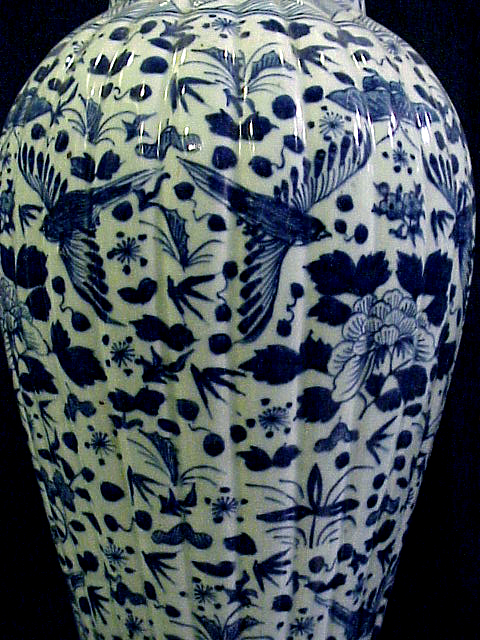*        *        *

A J   G a l l e r y     Tel: 212 585 2675   email: aj@ajgallery.com